# Edexcel A Level Economics A:复习笔记2.4.4 The Multiplier

### The Multiplier

• The multiplier ratio is the ratio of change in real income to the injection that created the change
• E.g. If the UK government injected an additional £5m into the economy through government spending and it resulted in an increase in real income of £15m, the value of the multiplier would be 3
• The multiplier process is based on the idea that one individual's spending is another individual's income
• An increase in consumption immediately increases AD
• Store owners who have benefitted from the extra consumption now have extra income
• They spend some of that income on goods/services
• Their expenditure on goods/services is now income for the next tier of individuals
• Due to the successive rounds of spending, the final increase in national income is much larger than the initial injection
• The size of the multiplier is entirely dependent on the size of leakages that occur during the process
• The higher the leakages the smaller the multiplier
• The initial injection shifts AD to the right
• The result of the multiplier process is that there is then a secondary movement of AD to the right which (if the multiplier were 2) may be double the initial movement
• The multiplier can also work in reverse when injections are reduced (downward multiplier effect)

### The Effects of Marginal Propensities on the Multiplier

• The 'marginal propensities' refer to the proportion of the next \$ earned that a consumer saves, consumes, is taxed, or purchases imports with
• Marginal propensities are calculated for economies and provide insights into how each additional \$ of income is allocated
• Sweden has a higher tendency to save than the USA
• Their marginal propensity to save is higher
• The USA, therefore, has a greater multiplier on any injections into the Circular Flow

An Explanation of The Marginal Propensities

 Marginal Propensity to Consume (MPC) Marginal Propensity to Save (MPS) Marginal Propensity to Tax (MPT) Marginal Propensity to Import (MPM) The proportion of additional income that is spent The proportion of additional income that is saved The proportion of additional income that is paid in tax The proportion of additional income that is spent on imports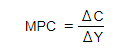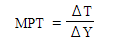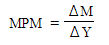### Calculating the Multiplier

• The value of the multiplier can be calculated one of two ways
• By focusing on the marginal propensity to consume (MPC)
• By focusing on the withdrawals that occur on each additional \$ of income (MPS + MPT + MPM)
1. Focussing on the MPC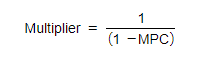2. Focusing on the Withdrawals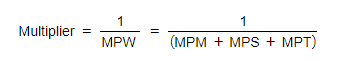#### Worked Example

An economy has the marginal propensity to save of 0.15, marginal propensity to tax of 0.20 and a marginal propensity to import of 0.15.

a) Calculate the size of the multiplier.

b) If the Government increases their infrastructure spending by £60m, calculate the total increase in GDP, assuming all other things remain equal.

Step 1: Insert the values into the withdrawal formula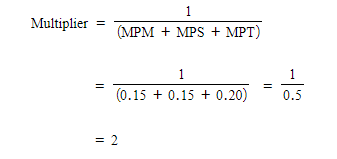Step 2: Multiply the injection by the multiplier

Impact on GDP = Injection x multiplier
= £60m x 2

= £120m

### Significance of the Multiplier in Shifting AD

• The greater the withdrawals, the smaller the value of the multiplier - and vice versa
• The greater the MPC, the greater the value of the multiplier - and vice versa
• Any change in one of the factors that impacts on disposable income, will change the multiplier
• If taxes increase the value of the multiplier reduces
• If interest rates increase, savings increase and consumption decreases and the multiplier reduces
• If exchange rates appreciate the level of imports will increase and the multiplier decreases
• If confidence in the economy increases consumption increases and the multiplier increases
• It is extremely useful for the Government to know the value of the multiplier
• They can use it to judge the likely economic growth caused by increased spending
• There is a time lag as it takes time for the successive rounds of income to work through the economy

#### Exam Tip

The final bullet point above mentions time lags. This is an excellent point to include in any evaluation on the effectiveness of the multiplier. It may take up to 18 months for the full multiplier effect to be seen & any change to consumer confidence during this period will impact the final outcome.